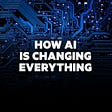# RL World

Reinforcement Learning is a very vast list of algorithms. Let's look at this structure of the Reinforcement world and try to understand it.

• MDP World:

This is the first division, Markov Decision Process(MDP). These are the algorithms that try to solve the problem, where we have an underlined vision of the world. There are states and rewards (which we get when we move from one state to another).

MDP provides a mathematical framework for modeling decision making in situations where outcomes are partly random and partly under the control of a decision maker.

• Bandit World

This is the 2nd type of division for the Reinforcement learning world. In this type of problem, there is no concept of States. There is essentially no such idea about moving from one state to another sequentially and gaining rewards. Here we are more concerned about learning the best decision to take when we are put in a certain type of condition. “Bandit” in “multi-armed bandits” comes from “one-armed bandit” machines used in a casino. In multi-armed bandit problems, the more you play the more you learn about the world, the more accurate we get about the decisions.

Real-world implementations could be a smarter AB Test: Instead of testing one thing on all populations, you will be making decisions based on a particular condition. You can show cat images to people who like cats, dog images to people who like dogs. Contextual Bandits can be used to optimize which algorithms to show on the website, for a higher click-through rate.

Bandits are divided into 2 types:

• Action Value methods and Gradient bandit Methods

MDP is further divided into Model-based and Model-free.

• Model-Based:

In these algorithms, we have some external knowledge about the environment. We can also say that here we have an idea about the rules of the game environment. Monte Carlo Methods are Model based methods. Example: Googles Deep mind — Alpha go and Model Zero uses Markov decision trees and a Model-based approach.

Advantage: we can create a decision tree from the beginning to the winning end which makes it interpretable.

Drawback: As the state-space and the action space begins to grow, the model becomes more and more complicated. It becomes computationally expensive.

• Model Free :

In this method we do not have an underlined structure of the environment. Learning everything about the environment from scratch. These models rely on Trials and error to update their knowledge of the environment. In this model we only have access to current state, reward and at maximum we can ask for the next reward.

Model Free are divided into 2 types:

• Value-based: In this method we try to find the best estimation of future value. Because at the end we need to maximize the rewards. We estimate the value function and the Policy is implicit.

ex. Q-learning, SARSA etc

• Policy-Based: In this method we try to find the best policy. Here we just want the agent to learn how to behave optimally in the environment. Theoretically, it is the best approach. There is No value functionand in this method, we just try to estimate the policy and optimize it.

ex. REINFORCE, Hill Climbing method etc

• Actor Critic Method: This method is a combination of the above 2 methods, known as Actor Critic. This method takes use of Value function in addition to the policy function. The value function is used to update the policy. In this method the “Critic” estimates the value function and “Actor” updates the policy distribution in direction of the Critic.

## Bellman Equation:

It is the basic building block of Reinforcement learning algorithms. It essentially helps in solving the policy and value functions.

It says that the value of a given state is equal to, selecting an action that maximizes the value of the state. So, the value for a given state is the sum of the reward we get from the current action (taken in the present state), in addition to the value of the state we end up in. γ is the discount factor, which is multiplied to this next state value.

## Deterministic vs Stochastic Policy

• Deterministic:
- It maps a state to an action. A single action is returned by the policy to be taken.
- They are used in deterministic environment. ex chess.
• Stochastic:
- In stochastic environment we have a probability distribution of the actions. There is a probability we will take a different action.
- It is used when an environemtn is uncertain

In some situations its better to have a stochastic policy because, if we learn just one fixed action in a particular state, the opponent will learn to exploit that state. Its better to have a uniform random policy.

• Behaviour Policy is the policy used by the agent to determine its actions in a given state.
• Target policy is the policy used by the agent to learn from rewards recieved for its actions, to update the Q-value.

## Value-Based are divided into 2 types:

• On- Policy : The target policy is same as the behavior policy.
• Off Policy : The target policy is different from the behavior policy.

The gradients help the model by giving it useful information on how the parameters should be changed in order to get better results.

## Policy-Based is divided into 2 types :

• Gradient free: This technique, the population of the parameter vector is mutated randomly, and the reward function is calculated. The parameter vector with the highest reward is recombined for the selection of the next parameter vector.
• Gradient-Based: In this technique we try to populate the parameter vector, by searching for the local maxima and calculate the reward for each of this maxima found. If the reward increases then we can either maintain the stepsize or decrease it, or else we increase the step size till we get higher rewards. This techniques relies on the optimization of the parametrized policies with respect to the expected return (which we want to maximize). This method targets the modeling and optimizing the policy directly.

## State Value Function

Vπ(s) is the expected value of following policy π (that is used endlessly) when the agent starts following it from this specific state (s).

## State Action Value Function ( Q function)

Qπ(s,a) expresses the expected value of first taking action (a) from state (s) and then following policy (π) from there on.

Difference: State Value function defined the goodness of a state, while a state-action value defines the goodness of an action in a state. In both these cases, we build a table, which can either be a value table or a Q table!!

This article tried to show some of the basic concepts used for implementing it in various Open AI’s Gym and Unity environment. These can be used for solving various real world problems as well. To look at these algorithms in detail and its implementations check out the Github

--

--

--

## More from Abhishek Dabas

Masters Student | Machine Learning | Artificial Intelligence | Causal Inference | Data Bias | Twitter: @adabhishekdabas

Love podcasts or audiobooks? Learn on the go with our new app.

## Getting started with Amazon SageMaker Studio Lab## Machine Learning: a digestible intro guide## Bayes Theorem & Confusion Matrix## Extractive Text Summarization for English, Hindi & Tamil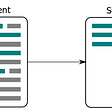## Synthetic tabular data for testing your applications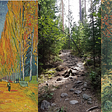## Singapore Flat Price Predictor## Easy explanation of Relational Deep Reinforcement Learning with real code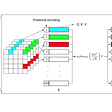## Understanding U-Net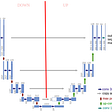## Abhishek Dabas

Masters Student | Machine Learning | Artificial Intelligence | Causal Inference | Data Bias | Twitter: @adabhishekdabas

## Can AI Predict Where You’ll be in 5 years?## The Impact of Racial Bias in Facial Recognition Technology## An Analytical Look Into Deep-Q Networks (DQNs)## Know How Artificial Intelligence is Changing the World